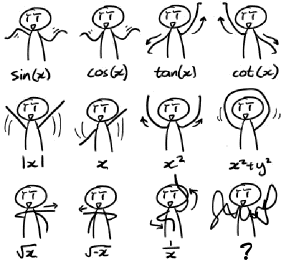## Graph studio

• Some of the most commonly used mathematical functions and their graphs.

## Basics

• There are certain descriptions which are often used when we talk about graphs.
Graph description Meaning
The graph is positive The y-values of the graph are positive.
The graph is negative The y-values of the graph are negative.
The graph is increasing As we move from left to right, the y-values on the graph climb.
The graph is decreasing As we move from left to right, the y-values on the graph drop.

## Dance moves

• You can remember these plots with some cool dancing moves!